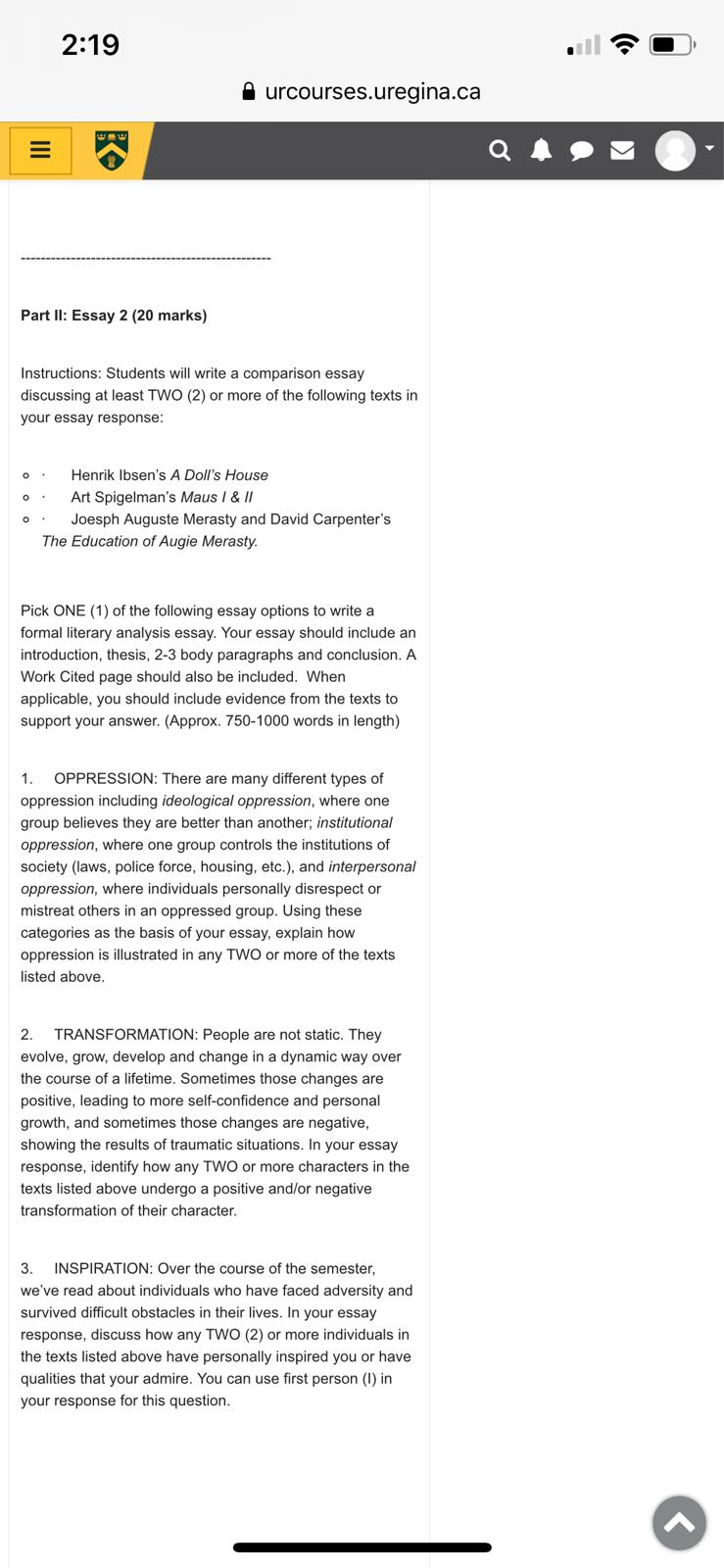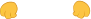### Ask This Question To Be Solved By Our ExpertsGet A+ Grade Solution Guaranteed##### Timotius AlbertMathematics
(/5)

Hire Me##### Darshan MaheshwariEducation
(/5)

Hire Me##### Laura LeighlandMathematics
(/5)

Hire Me(/5)

Hire Me

### The analysis of beams is an important topic in mechanical engineering. One type of beam considered is a cantilever beam in which one end of the beam is fixed and the other end of the beam is free

INSTRUCTIONS TO CANDIDATES

C++ Programming for Engineers
Programming Assignment: Beam Deflection
The analysis of beams is an important topic in mechanical engineering. One type of beam considered is a
cantilever beam in which one end of the beam is fixed and the other end of the beam is free.

When a weight, W, (or load) is applied the free end of the beam, the beam deflects or bends. The amount of
deflection, , on the free end of the beam depends on the beams loading conditions.
There are many types of loading conditions, but three types are considered here:
1) End load - A single load, W, is applied at the free end of the beam
2) Intermediate Load – A single load, W, is applied at point B (somewhere between the free end and the
fixed end)
3) Uniform Load – The load W is uniformly distributed along the length of the beam
W = total weight of load (N)
δ = deflection caused by the load (m)
x = distance from the free end of the beam (m)
l = L = length of the beam (m)
For a uniform load, W = wL [note: (N/m)(m)=N]
Page 2
The deflection for each beam can be calculated using the following relationships, assuming that the forces on
the beam are coplanar, the beam is in static equilibrium, and that the weight of the beam may be neglected.
where:
E = Young’s modulus of elasticity (N/m2)
I = moment of inertia (m4)
L = length of beam (m)
For the Intermediate Load, the following terms also apply:
a = distance from fixed end of beam to application of the load (m)
b = L – a
For the Uniform Load: Weight = (Weight Density)(Beam Length) or W = (w)(L)
Young’s modulus of elasticity varies with different types of materials. The following are typical values:
Material Young’s modulus (N/m2
)
Aluminum 6.90 x 10
10
Brass 11.7 x 10
10
Carbon Steel 20.5 x 10
10
Cast Iron 10.0 x 10
10
Oak 1.10 x 10
10
Stainless Steel 19.0 x 10
10
Titanium 11.4 x 10
10
Page 3
It will be assumed that the beam under consideration is an I-beam with the following dimensions:
H outer height of I - Beam (m)
h inner height of I - Beam (m)
B outer width of I - Beam (m)
b width of both flanges of I - Beam (m)
The moment of inertia, I,for the I - Beam can be calculated as follows :
Program Requirements:
Write a C++ program according to the following specifications
1. Display a brief description of the program.
the beam configuration and I-Beam shape with variables labeled to be sure that the user understands what
3. Ask the user to specify the type of material (give the user of menu of 7 material types listed above) and then
use the given value of E.
4. Ask the user for the beam dimensions (L, B, b, H, and h). Use a c++ function to find the moment of inertia.
5. If an end load or intermediate load is chosen, prompt the user to enter the weight of the load.
6. If a uniform load is selected, prompt the user to enter the weight density.
7. If an intermediate load is chosen, prompt the user to enter the value of b. (Note: This is the b on the
intermediate load diagram where a + b = L, not the b used in the beam dimension. You might want to
rename it.)
8. Create 3 c++functions to calculate δ for the three types of loading.
9. Prompt the user to specify the number of points, N (in addition to x = 0) to use in a table of x and values.
10. Neatly display the following:
A brief description of the program.
All input quantities (both variable and name) with units. Also display I.
Display a table of values of x and δ with an appropriate table heading (with units) and with all values
nicely aligned. Add lines to the table.

Page 4
Other important notes:
User prompts should include both symbol and name of variable and unit.
Use scientific notation with 3 digits after the decimal point for most results.
Check all inputs for errors. If any inputs are entered incorrectly, allow the user to correct them.
Error checks include:
o All beam dimensions, weights, and weight density must be positive
o H > h, B > b, L > a, and a > 0.
Give the user the option of re-running the program
Example: End Load, Carbon Steel I-Beam with B = 0.02 m, H = 0.04 m, b = 0.015 m, h = 0.03 m, W = 125 N,
L = 2 m, and N = 10. (Note: required formatting not used)
Test Cases
Run the program for the following test cases and turn in printouts of the results with your report.
Loading Material L (m) H (m) H (m) B (m) B (m) N W (N) W (N/m) a (m)
End Carbon
Steel
2 0.04 0.03 0.02 0.015 10 125
Intermediate Aluminum 10 0.05 0.04 0.025 0.02 20 500 5
Uniform Brass 0.5 0.06 0.04 0.03 0.024 15 300

## Related Questions

##### . The fundamental operations of create, read, update, and delete (CRUD) in either Python or Java

CS 340 Milestone One Guidelines and Rubric  Overview: For this assignment, you will implement the fundamental operations of create, read, update,

##### . Develop a program to emulate a purchase transaction at a retail store. This  program will have two classes, a LineItem class and a Transaction class

Retail Transaction Programming Project  Project Requirements:  Develop a program to emulate a purchase transaction at a retail store. This

##### . The following program contains five errors. Identify the errors and fix them

7COM1028   Secure Systems Programming   Referral Coursework: Secure

##### . Accepts the following from a user: Item Name Item Quantity Item Price Allows the user to create a file to store the sales receipt contents

Create a GUI program that:Accepts the following from a user:Item NameItem QuantityItem PriceAllows the user to create a file to store the sales receip

##### . The final project will encompass developing a web service using a software stack and implementing an industry-standard interface. Regardless of whether you choose to pursue application development goals as a pure developer or as a software engineer

CS 340 Final Project Guidelines and Rubric  Overview The final project will encompass developing a web service using a software stack and impleme